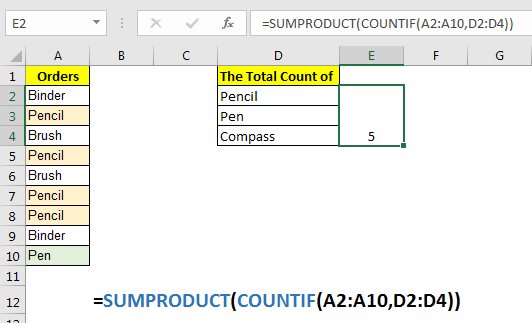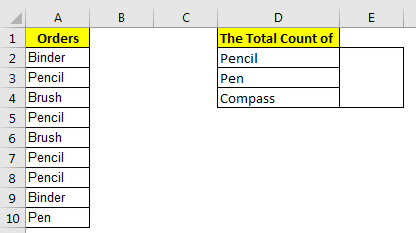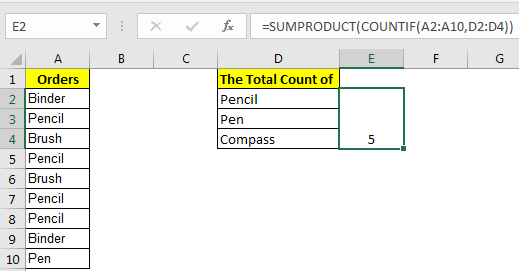# How to Count Cells That are Equal to One of Many Cells in ExcelSometimes we want to count cells that contain value defined in other cells. In that case, a simple COUNTIF and COUNTIFS function will not work. We can use a combination of SUMPRODUCT and COUNTIF function of Excel to achieve that.

Generic Formula to Count One of Many Cells

=SUMPRODUCT(COUNTIF(range,criteria)

Range: the range in which you want to match the criteria.

Criteria: the reference of values that you want to count in Range.

Let’s see an example to make things clear.

## Example: Count The Total of Pen and Pencils in  a List

Here I have this list in range A2:A10. In cell D2:D4 I have a list of items, whose total count is required.One way is to count each item separately using countif function and then add them up to get the total count of those items. For that I will be adding a helping row. But I don’t want to do that.

Another way is to use 2 countifs for each item and then use SUM function or addition operator. But if you have hundreds of items to get a count of then this method of counting will be useless.

Hence we use the above mentioned generic formula to get the total count of listed items.

Apply the above generic formula here to get the total count of each value.

=SUMPRODUCT(COUNTIF(A2:A10,D2:D4))

This returns 5. The pencil is found 4 times, Pen 1 time, and Compass is found 0 times.How it works?

Well this is quite simple. If you just write the COUNTIF(A2:A10,D2:D4) in a cell as an array formula, it will return the count of first value in range D2:D4. However, internally they are returning an array of counts for each item in D2:D4. {4;1;0} this is the array it is returning in the above example.
Now we just need to sum each the values. For that we used SUMPRODUCT function of Excel. It sums up the counts and gives us the total count of each value in given range.
You must be thinking, why not use SUM function. Well you can use it, but then you will need to use it as an array formula.
So yeah guys, this is how you get a count of multiple items using one simple excel formula. Let me know if you have any doubts regarding this function or any other function of excel. The comments section is all yours.

Related Articles:

Count Characters in a Cell in Excel | Learn how to count specific character in a cell or string.

Count total matches in two ranges in Excel | Learn how to count total matches in two ranges using SUMPROUDCT function.

SUMIFS using AND-OR logic | The SUMIFS can be used with OR logic too. The defualt logic SUMIFS uses is AND logic.

SUMPRODUCT with IF logic | Learn how to use SUMPRODUCT with IF logic without using IF function in the formula.

Popular Article:

50 Excel Shortcuts to Increase Your Productivity | Get faster at your task. These 50 shortcuts will make you work even faster on Excel.

The VLOOKUP Function in Excel | This is one of the most used and popular functions of excel that is used to lookup value from different ranges and sheets.

COUNTIF in Excel 2016 | Count values with conditions using this amazing function. You don't need filter your data to count specific value. Countif function is essential to prepare your dashboard.

How to Use SUMIF Function in Excel | This is another dashboard essential function. This helps you sum up values on specific conditions.

Terms and Conditions of use

The applications/code on this site are distributed as is and without warranties or liability. In no event shall the owner of the copyrights, or the authors of the applications/code be liable for any loss of profit, any problems or any damage resulting from the use or evaluation of the applications/code.